# Solve and Graph an Absolute Value Inequality

Nicole Conaway, Luke Winspur
• Author
Nicole Conaway

Nicole Conaway has taught Secondary Math and Science [subjects] for over 20 years. They have a Master's Degree in Environmental Science from the University of Michigan and a Bachelor's degree in Biology form Wayne State University. They also have a Professional Teaching Certificate from the State of Michigan.

• Instructor
Luke Winspur

Luke has taught high school algebra and geometry, college calculus, and has a master's degree in education.

Learn what absolute value inequalities are and how to solve absolute value inequalities. The lesson also covers the graphing of absolute value inequalities. Updated: 11/11/2021

Show

## Absolute Value Inequalities

An absolute value inequality is a mathematical expression of two unequal quantities which includes at least one absolute value.

### Absolute Value

The absolute value is the distance of a number from zero. Sometimes this is described as the magnitude. For example the absolute value of 5 is 5 and the absolute value of -5 is also 5. The symbol for absolute value is |n|, where n is a number. Absolute values may also be found for algebraic expressions. The number, variable, or expression within the absolute value symbol is called the argument. In general:

$$|n| = n$$

$$|-n| = n$$

Read: The absolute value of a number n is equal to n and the absolute value of -n is equal to n.

### Inequalities

An inequality is a mathematical expression stating that one quantity is greater than, greater than or equal to, less than, or less than or equal to another quantity, indicated by the following symbols: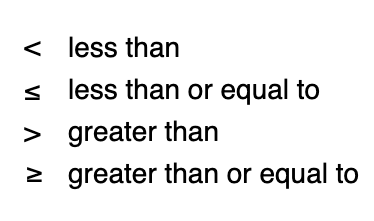A simple example of an absolute value inequality is $$|x| \geq 7$$

Following the definition and example above, the absolute value of x is equal to 7 if x is 7 or -7. So The absolute value of x is equal to or greater than 7 if x is any number greater than 7 or less than -7. This is because any number less than -7 will have an absolute value greater than 7.

The solution is written:

$$x\geq 7$$

or

$$x\leq - 7$$

This solution is read: X is equal to or greater than 7 OR x is less than or equal to -7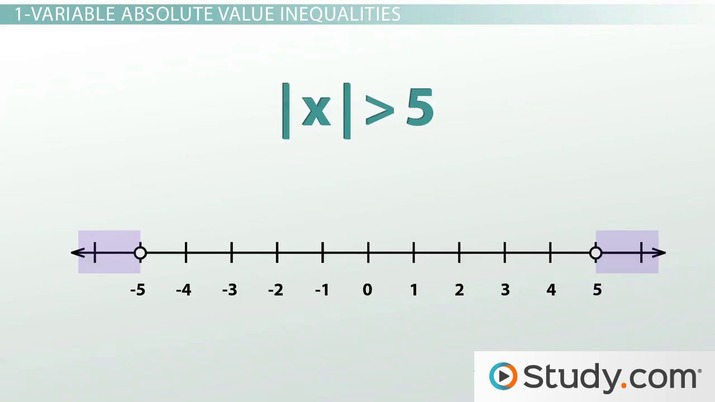An error occurred trying to load this video.

Try refreshing the page, or contact customer support.

Coming up next: Solving and Graphing Absolute Value Inequalities: Practice Problems

### You're on a roll. Keep up the good work!

Replay
Your next lesson will play in 10 seconds
• 0:24 Inequality/Absolute…
• 1:45 1-Variable Absolute Value
• 3:19 The Golden Rule
• 5:53 2-Variable Absolute Value
• 7:25 Summary
Save Save

Want to watch this again later?

Timeline
Autoplay
Autoplay
Speed Speed

## Absolute Value Inequality Rules

When solving absolute value inequalities there are some important rules to remember:

• Absolute value inequalities give us compound inequalities, a combination of two inequalities.
• Inequalities with a less than symbol have solutions that are intersections of two inequalities, described as "and"
• Inequalities with greater than symbols have solutions that are unions of two inequalities, described as "or"
• When solving inequalities, multiplication or division by a negative number reverses the direction of the inequality symbol
• Since absolute value is positive, an absolute value inequality set less than a negative number will have no solution.
• If the absolute value is greater than any negative number, then the solution is all real numbers

Use these steps to solve absolute value inequalities:

• First use inverse operations to isolate the absolute value on the left side.
• For 'greater than' inequalities, solve for the the inequality greater than the positive and flip the symbol and solve for the the negative value.

### Solving 'Greater Than' Absolute Value Inequalities

For example:

{eq}3|2-x|-5> 7 {/eq}

First,use inverse operations to isolate the absolute value: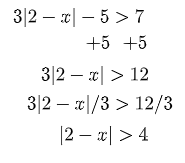Since this is a 'greater than' inequality, solve for the the inequality greater than the positive and flip the symbol and solve for the the negative value:

Solving for the positive option: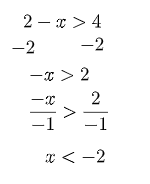Flip the inequality symbol and solve for the negative option: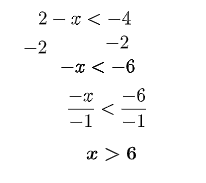The solution is X<-2 or x>6

### Solving a 'Less Than' Absolute Value Inequality

$$|3x+4|\leq25$$

This is a 'less than' absolute inequality so the solution will be an 'and' complex inequality. The values that make the inequality true must satisfy both conditions: they must be greater than the negative value AND less than the positive value. This type of inequality is written with the argument of the absolute value in the middle of the negative and positive options like this:

$$-25\leq |3x+4|\leq 25$$

Use inverse operations to solve for x: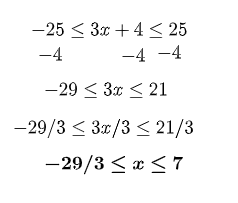This solution can be read "-29/3 is less than or equal to x and x is less than or equal to 7" or simply "-29/3 is less than or equal to x is less than or equal to 7"

Here is one more example: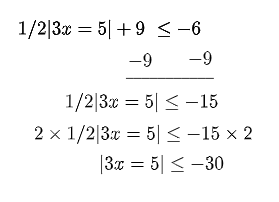In this case, when the absolute value expression is isolated, it is less than a negative value. Since absolute values are always positive, this inequality has no solution

## Graphing Absolute Value Inequalities

Graphing the solution to an absolute value inequality helps to make sense of the solution.

To unlock this lesson you must be a Study.com Member.

#### How do you solve absolute value inequalities?

Solve one dimensional absolute value inequalities algebraically. First, isolate the absolute value on the left using inverse operations. Solve for both the negative and the positive values. For "greater than" inequalities, flip the inequality symbol before solving for the negative value.

Solve two variable absolute value inequalities by graphing them. Graph the corresponding absolute value equation. Use a solid line if the inequality includes 'or equal to' or a broken line if it does not. Shade in the appropriate area above or below the line.

#### How does an absolute value symbol affect an inequality?

Because each number and its opposite (both positive and negative) have the same absolute value, the absolute value symbol means the inequality will have a two part solution, or a complex inequality. Finding the solution involves solving two inequalities, one for the negative value and one for the positive value.

### Register to view this lesson

Are you a student or a teacher?